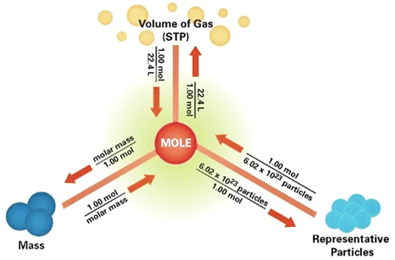Number of Moles Formula

Number of Moles Formulas - Avogadro’s constant

The mole is a measure or the base unit for the amount of substance present in the given sample. 1 mole is number which is equal to 6.022 x 1023 particles, also known as the Avogadro’s constant. These particles can be any type of species, e.g atoms, molecules, electrons, protons, neutron etc. In fact if we have a which contains approximately 6.022 x 1023 almonds, it can be said that we 1 mole of almonds in that particular box.

This number, 6.022 x 1023 (Avogadro’s constant) is actually defined based on the number of atoms present in 12g of 12C isotope of Carbon. Where 12gm is the molar mass of 12C or in other words the mass of 1 mole or 6.022 x 1023 atoms of 12C isotope of Carbon.

So in order to calculate the number of moles of any substance present in the sample, we simply divide the given weight of the substance by its molar mass.

Mathematically,
$n = \frac{m}{M}$
Where ’n’ is the number of moles, ‘m’ is the given mass and ‘M’ is the molar mass.
Here is a small map that can help you to relate number of moles, volume and mass of a substance.Example: How many moles are in 25.0 grams of water?
Solution:
In order to find the number of moles, we will have to use the following formula
$n = \frac{m}{M}$
Molar Mass, M, of H2O = 18.0 gm/mol
Given mass, m, of H2O = 25.0 gm
So, n = 25.0 (gm) / 18.0 (gm/mol)
Number of moles, n = 1.39 mol

Question: How many moles are in 3.4 x 1023 molecules of H2SO4?
Options:
(a) 0.82 moles
(b) 0.01 moles
(c) 5.56 moles
(d) 0.56 moles